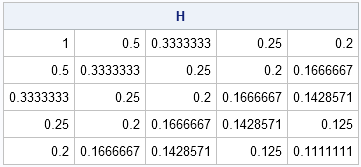The Hilbert matrix is the most famous ill-conditioned matrix in numerical linear algebra. It is often used in matrix computations to illustrate problems that arise when you compute with ill-conditioned matrices. The Hilbert matrix is symmetric and positive definite, properties that are often associated with "nice" and "tame" matrices.

The Hilbert matrix is definitely not tame, but it is nice in the sense that it is easy to construct. If H is a Hilbert matrix, then H[i,j] = 1 / (i+j-1). Notice that the elements are the reciprocals of integers and the matrix is banded along the diagonals where i+j is constant.

I have previously blogged about three ways to vectorize the construction of a structured matrix. The Hilbert matrix provides another example to practice using vector and matrix computations, rather than write DO loops in the SAS/IML language. Let's look at three ways to construct the Hilbert matrix.

### Method 1: Nested DO loops

The straightforward way to construct a Hilbert matrix is by writing nested iterative loops. This naive approach is not very efficient in a matrix-vector language such as SAS/IML, MATLAB, or R.

```proc iml; /* First attempt: nested DO loops. Inefficient */ n=5; H = j(n,n); do i = 1 to n; do j = i to n; H[i,j] = 1 / (i+j-1); H[j,i] = H[i,j]; end; end; print H;```In the preceding code, the loops take advantage of the symmetry of the Hilbert matrix. However, the method is inefficient because it loops over all rows and columns.

### Method 2: Constructing each row as a vector

In my previous article, I proposed identifying constant expressions within a loop and replacing the inner loop with a vector operation. That technique works with the Hilbert matrix. Notice that the expression j can be a vector that does not depend on the looping variable i. Consequently, you can rewrite the construction in the SAS/IML language as follows:

```/* Second attempt: One DO loop over rows, column numbers stored in a vector */ H = j(n,n); j = 1:n; do i = 1 to n; H[i, ] = 1 / (i+j-1); end;```

### Method 3: Use matrix operations

There are two kinds of arithmetic operations in linear algebra: matrix multiplication and elementwise operations. If you use elementwise division, the construction of the Hilbert matrix becomes a one-liner. However, for clarity I will use several intermediate matrices. You can use the ROW function in SAS/IML 12.3 software to construct the matrix that has the constant value i for every element of the ith row. Similarly, you can use the COL function to construct the matrix that is constant along columns. (If you do not have SAS/IML 12.3 or later, use the functions from a previous blog on structured matrices.)

For convenience, this final algorithm is written as a callable function, and I avoid using the ROW and COL functions in case some readers are running older versions of SAS/IML software. In the following function, matrices are placed in the denominator of a fraction and elementwise division is used to produce the matrix of reciprocals:

```/* Create an n x n Hilbert matrix. No DO loops. */ start Hilbert(n); i = repeat(T(1:n), 1, n); /* = row(H) */ j = repeat(1:n, n); /* = col(H) */ return( 1 / (i + j - 1) ); finish;   H = Hilbert(5);```

There you have it: A vectorized construction of the n x n Hilbert matrix. It is always good to practice vectorizing computations, and this exercise shows how to efficiently construct one of the most famous ill-conditioned matrices in numerical linear algebra.

ShareDistinguished Researcher in Computational Statistics

Rick Wicklin, PhD, is a distinguished researcher in computational statistics at SAS and is a principal developer of SAS/IML software. His areas of expertise include computational statistics, simulation, statistical graphics, and modern methods in statistical data analysis. Rick is author of the books Statistical Programming with SAS/IML Software and Simulating Data with SAS.

1.Rick, excellent tutorial on vectorizing operations. I didn't appreciate the power of using vectorized subscripts, so purely for fun to test it out:

start Dilbert(n);
i = repeat((1:n)`, 1, n);
j = repeat(1:n, n);
a = 1 / ( 1:2*n-1 );
return( shape(a[i+j-1],n,n) );
finish;

H = Dilbert(5);

•